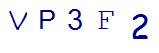# Back to School Basics: What Parents Need to Know about 6th Grade MathLast Updated on August 31, 2021 by Thinkster

Sending a kid off to 6th grade can bring many changes. For some, it is the start of middle school. For others, it is the end of elementary school. Most kids are in the throes of puberty and all of the social and emotional changes that this can bring. One thing that parents can prepare themselves for in this grade is math class. Math will be challenging this year. Here’s what you need to know about what your child will need to do for 6th grade math.

## Ratio, Statistics, and Probability

By 6th grade, students are ready to start learning some more abstract mathematical concepts. In this year, they will focus strongly on ratio, statistics, and probability. By the end of 6th grade, your students should be able to understand the basic concepts of ratios and use ratios to solve these problems. Teachers will also begin introducing the idea of statistical variability and will ask students to be able to summarize and describe distributions. These concepts can be challenging for parents who are not familiar with them, but help from Thinkster Math makes them easier to understand.

## Geometry

In geometry class, sixth graders should have a thorough knowledge of the basic shapes and prisms and the terminologies used to describe them. In this year, they will begin solving real-world problems involving area and volume and will also learn the concept of surface area. Geometry is one subject area where kids will get plenty of hands-on, real-world instruction.

## Expressions and Equations

Think 6th grade is too early for algebra? Think again. Actually, by 6th grade your child should have been doing quite a bit of algebra. Now they will extend that understanding and learn how to solve and reason with problems involving one variable, including both equations (with equals signs) and inequalities (with greater than or less than signs).  They will also be using dependent and independent variables.

## Basic Numbers and Operations

Multiplication, division, addition and subtraction do not disappear in sixth grade. It’s assumed by this grade that students will have a thorough handle on basic math facts. They will begin computing with them in a greater extent and will have to begin adding, subtracting, dividing and multiplying fractions and mixed numbers.

Common factors and multiples will be an important concept in this year of math. Students are going to need to find common factors and multiples of larger and larger numbers. They will also need to show a clear understanding of number systems. Negative numbers may also be introduced in this discussion.

## Connecting Math with Other Subjects

Because sixth graders should have basic math concepts down, this year they will be asked to perform math problems in other contexts. Their teachers may tie math into literature, science and history discussions. They will be asked to derive and solve equations from day-to-day activities. They may be asked to use pictures to create a numeric expression.

If these concepts seem above your head, you are not alone. Many parents struggle to help their kids with 6th grade math. That’s where Thinkster Math can help. Thinkster gives you the help of seasoned teachers in your child’s hands on a tablet. It uses game-like interface to keep kids engaged and learning without feeling pressure and provides instruction at the point of learning for more effectiveness. This online tutoring method can make complex 6th grade math concepts a breeze.

SummaryArticle Name
Back to School Basics: What Parents Need to Know about 6th Grade Math
Description
Author
Publisher Name
Thinkster Math
Publisher Logo

## Subscribe to Thinkster Blog## Recommended Articles### Unlocking the Code: Teaching Mathematical Symbols and Their Meaning

Knowledge of mathematical symbols is a foundational skill that empowers students to engage with and excel i...